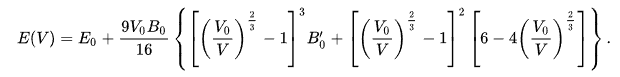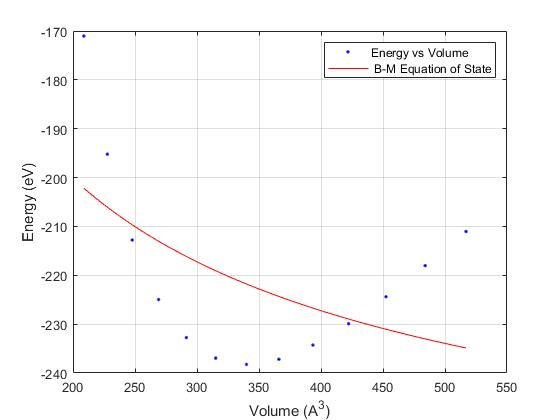# How to get fine fitting of B-M equation with high nonliearity?

12 views (last 30 days)
L world on 5 Aug 2022
Commented: Rena Berman on 23 Aug 2022
I have tried to fit Birch's equation like below:but I could not get fine fit..This is my code.
clc
clear all
data=[208.75 -171.04204447
227.72 -195.21101066
247.80 -212.78884133
269.03 -224.97316547
291.44 -232.75405432
315.06 -236.95077221
339.92 -238.24362657
366.05 -237.19727153
393.50 -234.28678793
422.28 -229.90809458
452.43 -224.39439701
483.98 -218.02503783
516.97 -211.03510699
];
V=data(:,1);
E=data(:,2);
ft=fittype( 'E0+9/16*(V0*B0)*Bp*((V0/V)^(2/3)-1)^3+(((V0/V)^(2/3)-1)^2)*(6-4*((V0/V)^(2/3)))', 'independent', {'V'}, 'dependent', {'E'}','coefficients',{'E0','V0','B0','Bp'})
[fitresult, gof] = fit( V, E, ft,'StartPoint',[-1 1 4 1])%, opts );
figure( 'Name', ' B-M' );
h = plot( fitresult, V, E );
legend( h, 'Energy vs Volume', ' B-M Equation of State', 'Location', 'NorthEast' );
xlabel('Volume (A^3)');
ylabel('Energy (eV)');
grid on
Please check my code for good fitting and wether there is error for a fit.
Best regards
Rena Berman on 23 Aug 2022
(Answers Dev) Restored edit